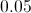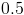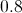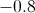# High School Math : Understanding Z-Scores

## Example Questions

### Example Question #3 : How To Find Z Scores For A Data Set

What is the-score for a value of 115 when the mean of the population is 103 and the standard deviation is 8?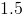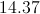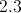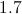Explanation:-score indicates whether a particular value is typical for a population or data set.  The closer the-score is to 0, the closer the value is to the mean of the population and the more typical it is.  The-score is calculated by subtracting the mean of a population from the particular value in question, then dividing the result by the population's standard deviation.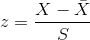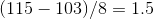### Example Question #1 : Graphing Data

A value has a-score of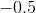.  The value is . . .

below the population mean

two standard deviations from the population mean

the same as the population mean

one standard deviation from the population mean

above the population mean

below the population mean

Explanation:

The-score indicates how close a particular value is to the population mean and whether the value is above or below the mean.  A positive-score is always above the mean and a negative-score is always below it.  Here, we know the value is below the mean because we have a negative-score.

### Example Question #1 : How To Find Z Scores For A Data Set

A population of values has a mean of 43 and a standard deviation of 12.  One of the values in the population is 49.  What is the Z-score for that value?